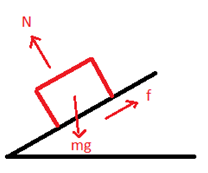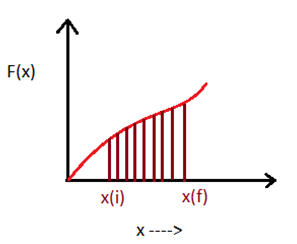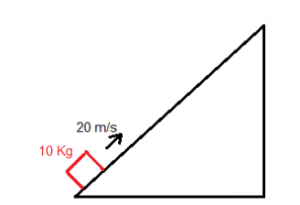# Work Energy Theorem And Its Application

## Introduction

We already discussed in the previous article (link here) that there is some relation between work done and energy. Now we will see the theorem that relates them. According to this theorem, the net work done on a body is equal to change in kinetic energy of the body. This is known as Work-Energy Theorem. It can be represented as

Kf – Ki = W

Where Kf = Final kinetic energy

Ki = Initial kinetic energy

W = net work done

So the above equation follows the law of conservation of energy according to which we can only transfer energy from one form to another. Also here the work done is the work done by all forces acting on the body like gravity, friction, external force etc. For example, consider the following figure,According to Work energy theorem,

Work done by all the forces = Change in Kinetic Energy

Wg + WN + Wf  =Kf – Ki

Where Wg = work done by gravity

WN = work done by a normal force

Wf = work done by friction

Kf = final kinetic energy

Ki = initial kinetic energy

### Work done by a constant force

A constant force will produce constant acceleration. Let the acceleration be ‘a’.

From equation of motion,

v2 = u2 + 2as

2as = v2 – u2

Multiplying both side with mass ‘m’

(ma).s = $$\frac {(mv^2 – mu^2)}{2}$$

F.s = $$\frac {(mv^2 – mu^2)}{2}$$

Comparing the above equation we get,

Work done by force (F) = F.s

Where ‘s’ is the displacement of the body.

### Work done by Non-Uniform Force

Now the equation,

W = F.ds

This is only valid when force remains constant throughout the displacement. Suppose we have a force represented below,For these kinds of forces, we can assume that force remains constant for a very small displacement and then integrate that from initial position to final position.

W = $$\int^{x_f}_{x_i} F(x) dx$$

This is work done by a variable force. A graphical approach to this would be finding the area between F(x) and x from $$x_i$$  to $$x_f$$ .The shaded portion represents the work done by force F(x).

## Frequently Asked Questions – FAQs

We will discuss some of the most common questions on this topic.

1. What is the statement of work energy theorem?

According to work energy theorem, the work done by net force on a body is equal to change in kinetic energy.$$\int~\overrightarrow{F}.d\overrightarrow{r}$$ = $$K_f~-~K_i$$

$$\int~\overrightarrow{F}.d\overrightarrow{r}$$ = $$K_f~-~K_i$$

2. How can we prove work energy theorem?

We know, $$K$$ =  $$\frac{1}{2}mv^2$$

Differentiating with respect to time we get,

$$\frac{dK}{dt}$$ = $$mv. \frac{dv}{dt}$$

If the resultant force makes some angle with the velocity, then

$$\frac{dK}{dt}$$ = $$Fv~cos\theta$$

$$\Rightarrow~dK$$ = $$\overrightarrow{F}.d\overrightarrow{r}$$

3. Steps to approach problems on work energy theorem?

Following steps should be considered:

Step-1: Draw the FBD of the object, thus identifying the forces operating on the object.

Step-2: Finding the initial and final kinetic energy.

Step-3: Equating the values according to the theorem.

4. How can we efficiently use this theorem?

Ans – The problems in which we have to calculate work done by a particular force, we can either to it using the equation of work, or directly apply this theorem to get the result. For example, consider the following problem:

A block of mass $$10~ kg$$ starts moving up the incline with $$20 m/s$$. It reaches the top and comes back to its initial position and stops. What is the work done by friction in the whole process?If we want to use the formula of work then we need the coefficient of friction to calculate frictional force. But that is not given. So let’s try to use work-energy theorem. Forces acting on the block are gravity, normal reaction, and frictional force. So,

$$W_f~+~W_N~+~W_g$$ = $$K_f~-~K_i$$

Here, $$W_N$$ is zero as force is always perpendicular to displacement. Being a conservative force $$W_g$$ is zero as the body returns to its initial position. Also,

$$K_i$$ = $$\frac{1}{2}~\times~10~\times~400$$ = $$2000 ~J$$

$$K_f$$ = $$0$$

$$W_f$$ = $$-2000~ J$$

Hence the work done by friction is negative.

Stay tuned with BYJU’S for more such interesting articles. Also, register to “BYJU’S – The Learning App” for loads of interactive, engaging Physics-related videos and an unlimited academic assist.

Test Your Knowledge On Work Energy Theorem And Its Application!